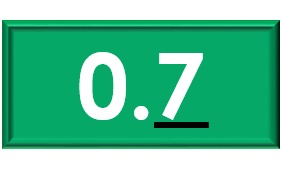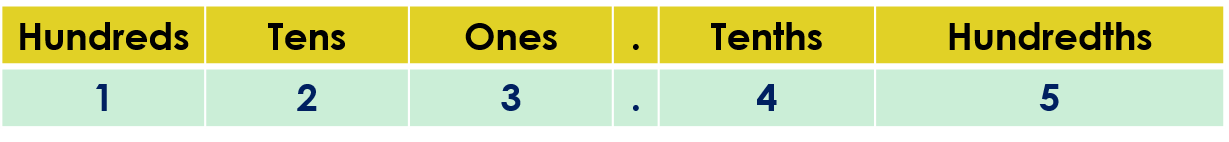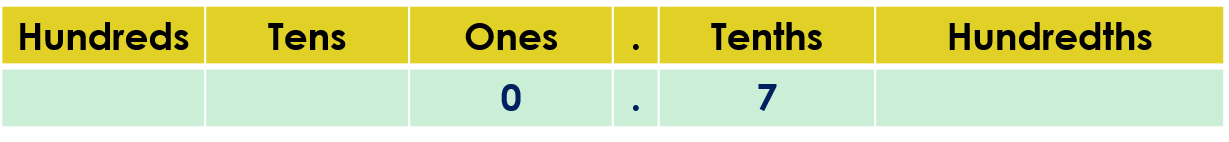1
visibility

What is the value of the underlined digit in the given number?• A

70

• B

7

• C

0.7

• D

0

The correct answer is "0.7".

Please Note:

Place value can be explained as the value represented by a digit in a number on the basis of its position in the number.

For example: Given the number 123.45.
Find the values of the digits 2 and 5.

But, the number 123.45 can be represented in the place value chart as:The value of the digit 2 is 2 tens or 20.
The value of the digit 5 is 5 hundredths or 0.05.

Solution:

Write the given number in the place value chart and identify the place of the underlined digit.To identify the place of the digit 7 in the number 0.7, we look for the position of 7 in the place value chart.

The digit 7 is in the tenths place.

So, the value of the digit 7 is 7 tenths or 0.7.